Maths-
General
Easy

Question

# Find the area of a triangle, two sides of which are 18 cm and 10 cm and the perimeter is 42 cm.Hint:

## The correct answer is: 69.65 cm2

### Let third side be xIt is given that Perimeter of triangle = 42 cm18 + 10 + x = 42x = 42 – 28 = 14So, length of third side is 14 cmUsing Heron’s formula,Area of triangle =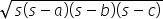where s =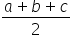s =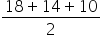= 21Area of triangle =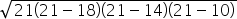=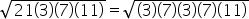= 21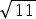cm2(= 3.31)= 69.65 cm2#### With Turito Foundation.#### Get an Expert Advice From Turito.